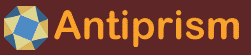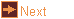# wythoff - Wythoff-style constructions

 Usage    |    Examples    |    Notes

## Usage

```

Usage: wythoff [options] [input_file]

Read a file in OFF format and apply a specified pattern to generate polygon
tiles. The polyhedron faces are divided by a 'meta' operation into triangles
each having vertices which are a vertex V, edge centre E and face centre F.
A start point is positioned on one of these triangles, the next point is
found by using the pattern to step between triangles, leading to a circuit.
If input_file is not given the program reads from standard input.

Options
-h,--help this help message (run 'off_util -H help' for general help)
--version version information
-p <pat>  pattern in form: [Coords0:Coords1:...]Path0,Path1...
Coordinates are barycentric, in form aVbEcF:
VEF element letters, and a,b,c are barycentric coordinates
corresponding to the following element letter. Ommiting a
an element letter and coordinate sets the coordinate to zero.
Ommitting just the coordinate sets the coordinate to 1. E.g
V = (1,0,0), VE = (1,1,0), V2E3F = (1,2,3)
Paths are in the form: TrisPidx0Move0Pidx1Move1...
Tris: one of +-* (default +) indicating that paths should
start for positive, negative or both kinds of triangles.
Pidx: an index number of a point from the coordinates list
Move: an operation for stepping to the next triangle, given
as a series of characters from the following:
_     - no move, stay on the same triangle
v,e,f - step over side opposite V,E,F
V,E,F - step two trianglesi, rotating about V,E,F,
according to: V=ef, E=fv, F=ve
Paths can start or end with either a move or a point
-c <op>   Conway polyhedron notation operator, or 'list' to list all
available operators with their corresponding patterns
-R        reverse pattern, exchanges the signs of the start triangles
-r <elms> relabel pattern, exactly three letters VEF written in any order
e.g. EFV relabels the pattern as V->E,v->e,E->F,e->f,F->V,f->v
-M        input geometry is a 'meta' tiling, don't apply meta operation
-C <mthd> tile colouring method, optionally followed by ':' and
point colouring method.
Tile colouring methods
none - do not colour tiles
index - use the path index, and apply colormap (default)
association - colour tiles using corresponding base element
colour, optionally followed by a comma and a letter from V,
E, F, or a colour, to use for all local tiles (default: F)
Point colouring methods (default: use tile coloring method)
none - do not colour points
index - use the point index, and apply colormap
association - colour points using corresponding base element
colour, optionally followed by a comma and a letter from V,
E, F, or a colour, to use for all local points (default: F)
component - colour by non-zero components, V=1, E=2, F=4,
V&E=3, E&F=6, V&F=5, V&E&F=7, and apply colormap
weight - colour using barycentric coordinates as RGB
-m <maps> a comma separated list of colour maps used to transform colour
indexes (default: spread), a part consisting of letters from
v, e, f, selects the element types to apply the map list to
-u        output only one example of each type of tile (one per path)
-f <ht>   lift the face centres by this height
-q        quiet, don't print report
-o <file> write output to file (default: write to standard output)

```

## Examples

List the Conway operators for lots of examples of the constructive notation
```wythoff -c list
```

Wythoff equivalents to |pqr, p|qr, pq|r, pqr|, for (2 3 5). The base model schwarz_2_3_5p is already the 'meta' triangle tiling so use option -M.
```wythoff -M -p [VEF]0V,0E,0F,0V0E0F schwarz_2_3_5p | antiview
wythoff -M -p [V]0E,0F schwarz_2_3_5p | antiview
wythoff -M -p [VE]0V,0E,0v0e schwarz_2_3_5p | antiview
wythoff -M -p [VEF]0v0e,0e0f,0f0v schwarz_2_3_5p | antiview
```

By default a polyhedron is processed by the Conway 'meta' operation before applying the constructive notation. Here is the equivalent of applying Conway notation 'ambo' to a cube
```wythoff -p [E]0V,0F cube | antiview
```

If the base polyhedron is already like a meta tiling, i.e. 2-colourable triangle faces and even order vertices, then it may be used as-is by specifying -M. For example, the following unitle2d triangle-tiled torus is like a 'meta' tiling, but does not correspond to a polyhedron, and the snub operation is applied to it
```unitile2d -s t -w 8 -l 24 2 | wythoff -c s -M | antiview
```

Models may be coloured using the colours from the base model, in this example the faces of a dodecahedron are coloured randomly, the model is processed with Conway operations p and o4, and coloured by element association. The final model shows patches of colour corresponding to the original coloured faces.
```off_color -f U dod | wythoff -c p -C a | wythoff -c o_4 -C a | off_util -S | antiview -x ev
```

The associated element colouring associates local tiles to the corresponding face element by default, but they can also be associated with vertices, edges or faces, or coloured with a colour
```off_color cube -v S,Ci -e grey | wythoff -c o_4 -C a,v | antiview
off_color cube -e S,Ci | wythoff -c o_4 -C a,e | antiview
off_color cube -f S,Ci -e grey | wythoff -c o_4 -C a,f | antiview
off_color cube -f S,Ci -e grey | wythoff -c o_4 -C a,white | antiview
```

## Notes

wythoff expands on the ideas behind the Wythoff symbol to create a specified tiling pattern from any suitable triangular tiling.

Triangle tilings are created, by default, from the input model by the Conway 'meta' operation. This consists of all triangles that join a face centre to one of its vertices and a neighbouring edge centre. With regard to starting triangles, the meta triangle (Vn, En, F) is labelled -/black, and the meta triangle (Vn+1, En, F) is labelled +/white. Alternatively, with option -M the input will be considered to be a suitable triangle tiling, and must be 2-colourable and all the vertices of even order.

The output tiling will not necesarily be planar, or have equal edge lengths. The output of 'spherical' tilings can be processed with the canonical program.

Several colouring methods are available for the final model with option -C. For points or tiles, index will colour by the position of an element in the pattern, and association will colour final elements with colours taken from elements in the base model (see below). Points can also be coloured with weight, using their normalised barycentric coordinates as RGB, and component, using the base elements that are involved in their pattern coordinates: V=0/red, E=1/green, F=2/blue, VE=3/yellow, EF=4/cyan, FE=5/magenta, VEF=6/grey. Set alternative colormaps with option -m, and use -m null to retain the original colour index numbers.

#### Comparison with corresponding Conway notation operators

Conway notation is applied to polyhedra, where vertices, edges and faces are clearly distinguishable. Constructive notation is applied to triangle tilings, derived from polyhedra by the Conway meta operation, and in these triangle tilings the three "element" types are equivalent.

Some Conway operators produce an edge with the same centre as an original edge. A corresponding constructive notation operation must produce a digon for this edge, because it is a polygon that wraps the element, and not just the edge of an adjoining face.

As a consequence, the Conway seed corresponds to a constructive notation 'seed' operator which, when applied to a polyhedron, generates the original polyhedron, but with digons along the edges.

As element types on the base tiling are equivalent, constructive notation operations that can be transformed into each other by permuting the element types can also be considered equivalent. Some equivalent Conway notation operators expressed in constructive notation are: seed/dual/ambo, truncate/zip/expand, kis/needle/subdivide. Some operators are symmetric with respect to the element types, for example snub, meta and bevel.

#### Element association

When the pattern paths are repeated to generate final faces, these faces may be associated with elements in the base model. A path may: cycle a base vertex, edge centre or face centre; not cycle(the path is the whole face); repeat by reflection; or "wander" around the model (the path doesn't cycle locally). The behaviour can be determined from the path specifier.

To analyse a path, write its reflections as a sequence, and remove sequential repeated reflections. It will then be in the form (step)association(step_back), where step is a series of mirrors (maybe none) and step_back is the same series of mirrors in reverse. The series of mirrors in association (which may be none) then determines the kind of element associated with the path, for eample it may be a rotation about an element of a type, etc. The step is the reflections to move from from the starting meta triangle to the meta triangle that contains the associated element.

As a example, the patternd fo truncate is [VE]0V0E,0V,0E. Write down the first path, remove the point specifiers, convert to reflections, and remove sequential repeats: 0V0E -> VE = (ef)(fv) = effv = ev this gives no step, and the association is ev, which is a rotation about a face centre (a reverse direction F). The final face will therefore cycle the face element of its starting meta triangle. Similarly, the second path will cycle the vertex element on the starting meta triangle, and the third path will cycle the edge element.

For the purposes of colouring, vertices are associated with base elements as follows: a final vertex is associated with a coinciding base vertex, or an edge if it coincides with an edge centre, otherwise the face element of the meta triangle it belongs to.

#### Repalcement for to_nfold

The to_nfold program has some similarities with wythoff, and automatically infers the tiling pattern from the base model, but only works for cyclic models. Its handing of oblique polygons that wind the axis will not allow it to produce series like the crossed antiprisms.

On the other hand, there is no way for a wythoff pattern to specify a cycle like 0(N/2 steps of)V1(N/2-1 steps of)V0V, which could draw a triangle that cycles any axis at an oblique angle, because the steps that need to be specified are relative to the N of the axis.

A solution is to produce a wythoff pattern that is parameterised by N/D, and apply this to a schwarz_N_2_2p meta tiling. The following example shows how this technique can be applied to generate the crossed antiprisms.

Display a 5/2 crossed antiprism, along with a suitable schwarz tiling to represent the base meta-tiling

```polygon ant 5/3 | off_trans -S 2 | antiview - schwarz_5_2_2p
```

Work out a pattern that generates the crossed antiprism for the meta-tiling (the -r option is to make the vertex elements lie on the axis, which is perhaps easier to follow)
```wythoff -M schwarz_5_2_2p -r FVE -p [VEF]0VV,0VVE0EVVE0EV | antiview
```

The first cycle is a fixed number of V's for the polygon step (2): VV = (D)V In the second cycle: the first step is relative to N and D (slant "down") VVE = ((N-D+1)/2)V ; the second step is relative to D (horizontal) EVVE = E(D)VE ; the third step is relative to N and D (slant "up") EV = E((N-D-1)/2)V .

The full parametrised pattern is:

[VEF]0(D)V,0((N-D+1)/2)VE0E(D)VE,0((N-D-1)/2)V

To generate an 11/4 crossed antiprism, which has Dv symmetry like the 5/2 crossed antiprism, N=11 and D=4, giving the following pattern

[VEF]0(4)V,0((11-4+1)/2)VE0E(4)VE,0((11-4-1)/2)V = [VEF]0VVVV,0VVVVE0EVVVVE0VVV

Construct the 11/4 crossed antiprism

```wythoff -M schwarz_11_2_2p -r FVE -p [VEF]0VVVV,0VVVVE0EVVVVE0EVVV | antiview
```

For crossed antiprisms with Dh symmetry the parameterised pattern is different, and could be determined to be, e.g.

[VF]0(D)V,0((N-D)/2+1)VE0E(D)VE0E((N-D)/2-1)V

Here is the pattern for the 13/5 crossed antiprism, found by plugging N=13 and D=5 into the parametrised pattern above

[VF]0(5),0(13-5)/2 + 1)VE0E(5)VE0E((13-5)/2 - 1)V = [VF]0VVVVV,0VVVVVE0EVVVVVE0EVVV

Construct the 13/5 crossed antiprism

```  wythoff -M schwarz_13_2_2p -r FVE -p [VF]0VVVVV,0VVVVVE0EVVVVVE0EVVV | antiview
```

#### Replacement for twist program

The twist program created a model connected like a twisted struct zig-zag tensegrity. The wythoff program can create equivalently connected models with the following pattern.
```wythoff -p [4V2E8F]0feEV0fe0E0V ico | antiview
```